Next: Retarded potentials Up: Relativity and electromagnetism Previous: The potential 4-vector

## Gauge invariance

The electric and magnetic fields are obtained from the vector and scalar potentials according to the prescription (see Sect. 4.3)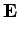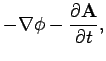(1443)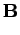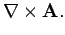(1444)

These fields are important, because they determine the electromagnetic forces exerted on charged particles. Note that the above prescription does not uniquely determine the two potentials. It is possible to make the following transformation, known as a gauge transformation, which leaves the fields unaltered (see Sect. 4.4):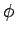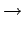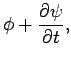(1445)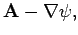(1446)

where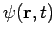is a general scalar field. It is necessary to adopt some form of convention, generally known as a gauge condition, to fully specify the two potentials. In fact, there is only one gauge condition which is consistent with Eqs. (1436). This is the Lorentz gauge condition,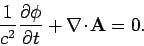(1447)

Note that this condition can be written in the Lorentz invariant form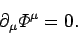(1448)

This implies that if the Lorentz gauge holds in one particular inertial frame then it automatically holds in all other inertial frames. A general gauge transformation can be written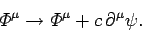(1449)

Note that even after the Lorentz gauge has been adopted, the potentials are undetermined to a gauge transformation using a scalar field,, which satisfies the sourceless wave equation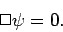(1450)

However, if we adopt sensible boundary conditions in both space and time then the only solution to the above equation is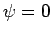.Next: Retarded potentials Up: Relativity and electromagnetism Previous: The potential 4-vector
Richard Fitzpatrick 2006-02-02